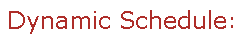Visit Minerva Class Schedule for course dates & times.

# ECSE 205 Probability and Statistics for Engineers (3 credits)

Offered by: Electrical & Computer Engr (Faculty of Engineering)

### Overview

Electrical Engineering : Probability: basic probability model, conditional probability, Bayes rule, random variables and vectors, distribution and density functions, common distributions in engineering, expectation, moments, independence, laws of large numbers, central limit theorem. Statistics: descriptive measures of engineering data, sampling distributions, estimation of mean and variance, confidence intervals, hypothesis testing, linear regression.

Terms: Fall 2022, Winter 2023

Instructors: Leib, Harry (Fall) Leib, Harry (Winter)

• Not open to students who have taken ECSE 305.

• (3-2-4)